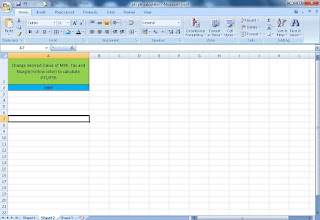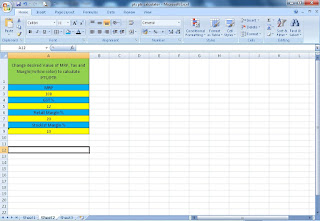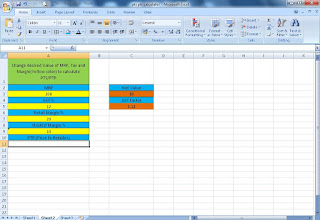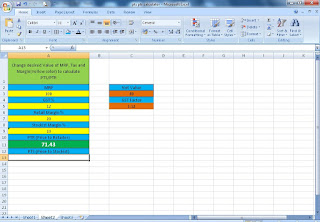### How to calculate Price to retailer (PTR) and Price to Stockist (PTS) from any given Maximum Retail Price (MRP)?

You have fixed Maximum Retail price for your product. You have also fixed profit margin, you want to give to retailers, stockists and other distribution channel business partners. But you don’t have knowledge how you can calculate at what price you will bill to stockist and stockist will be to retailers. Then this article is going to be very useful for you...

In this article, we will calculate price to stockist (PTS) and Price to Retailers (PTR) with any given MRP and margin percentage. Also we will provide a PTR/PTS calculator along with how you can make your own PTR/PTS calculator in excel format and we will also provide link to download PTR/PTS calculator in excel.

Check related article: profit margin in pharmaceutical industry (Manufacturer to retailers)

Have a look at important definitions related to this topic:

Distribution Channel: Distribution channel is a group of businesses involved in process of delivery a product/service to its end user from manufacturer. Check distribution channel set-up detail here

Maximum Retail Price (MRP): Maximum retail price (MRP) is a maximum value that can be paid by an end user for purchasing a product or using a service.

Price to Retailer (PTR): Price to retailer is price at which a stockist/manufacturer sells goods/services to retailers. It is the price which is fixed by deducting Tax and profit margin of retail from Maximum retail price.

Price to Stockist (PTS): Price to stockist is the price at which a manufacturer/CnF sells goods/services to stockist for distribution to retailers. PTS is generally calculated by deducting stockist margin from PTR.

## Now can to the point How to calculate PTR and PTS?

Here we are discussing most common method for calculating PTR/PTS. Values you need before calculating PTR and PTS.
• Maximum Retail Price
• GST value (5%, 12%, 18%, 28%)
• Retailer Margin
• Stockist Margin
Suppose
• MRP = 100/-
• GST value = 12%
• Retailer Margin = 20%
• Stockist Margin = 10%
First you need to deduct retailer margin from MRP. This will give a net value that in other words we can say PTR including GST like below:

Formula used will be MRP*(1-Retailer Margin %)
Net value = 100*(1-20%) = 80/-

### PTR Calculation:

Now calculate Price to retailer. Price to retailer (PTR) will be calculated by dividing GST value from above net value. For that you need to first calculate GST factor value. Formula for GST factor calculation is finding below:

GST Factor = (100+GST)/100
GST Factor value = (100+12)/100 = 112/100 = 1.12
PTR calculation formula = Net Value / GST Factor = 80/1.12 = 71.43/-

### PTS Calculation:

Price to stockist will be calculated by deducting stockist margin percentage from retailer price. Formula for calculating price to stockist (PTS) is like:
PTS calculation formula: PTR*(1 – Stockist Margin %) = 71.43*(1-10%) = 64.29/-

### Other Calculations:

If you want to calculate other calculations like Cnf price, sub stockist price etc then you can use formula like describe above. Suppose CnF margin is 6% then it will be calculate by below formula:
CnF (Carrying & Forwarding Agent Price) = PTS*(1 – CnF margin %) = 64.29*(1-6%) = 60.43/-

## How to prepare a PTR/PTS calculator in excel as embed above?

Open excel
At first row (Column A) write: Change desired Value of MRP, Tax and Margin(Yellow color) to calculate PTS/PTR as given below in image:Third row (Column A) is for MRP valueFourth Row (Column A) indicating GSTFifth Row (Column A) is for GST valueSixth row (Column A) indicating Retailer MarginSeventh row (Column A) is for retailer margin valueEight row (Column A) indicating Stockist marginNinth row (Column A) is for stockist margin valueNow in column C (Row 3) indicate Net value in a cell as shown in picture belowIn below net value cell, add a formula: = A3*(1-A7%) which will give net valueBelow this cell indicate GST FactorBelow GST indicator cell, add formula = (100+A5)/100 which will give GST factor valueNow come to tenth row (Column A). Write PTR (Price to Retailer)In eleventh row (column A), write formula: =C3/C5Twelfth row (Column A) indicating Stockist marginIn thirteenth row (column A), write formula: =A11*(1-A9%)## Conclusion:

By this way you can make, your own PTR/PTS calculator in excel format and use it for calculating your all product PTR/PTS values. You can also download PTS/PTR calculator from here

## Another Method to calculate PTR/PTS:

How to calculate?

There is a formula to calculate PTS and PTR. We can calculate it with GST or without GST. When you have GST number then it is required to calculate PTS/PTR without GST and add GST after invoicing value. If you don’t have GST number then it is required to calculate PTS/PTR with GST and can’t add GST at invoice.

Formula is Value multiply by 100 divided by 100 plus percentage of margin i.e. Value*100/(100+Percentage).

Here percentage may be GST, Stockist margin, Retailer Margin, CnF margin etc.

Value may be MRP in case of PTR calculation, PTR in case of PTS calculation etc.

Suppose we have a product having

MRP of 95 rs
GST 12%
Retailer Margin 20%
Stockist Margin 10%
Calculation of Price to Retailer:
Formula is Value*100/ (100+Percentage)
PTR = MRP*100 / (100+Retailer Margin) = 95*100/(100+20) = 9500/120 = 79.17/-
This PTR is included of GST. Now calculate it without GST.
PTR (Without GST) = PTR (With GST)*100 (100+GST) = 79.17*100 (100+12) = 79.17/112 = 70.69/-

Calculation of Price to Stockist: Formula is Value*100 / (100+Percentage)
PTS = PTR * 100 / (100+Stockist Margin) = 79.17*100 / (100+10) = 7917/110 = 71.97/-
This PTS is included of GST. Now calculate it without GST.
PTS (Without GST) = PTS (With GST) * 100 / (100+GST) = 71.97*100 / (100+12) = 7197/112 = 64.26/-

Hope this information is helpful to you...

Name

Email *

Message *